# Crossing numbers

## The Crossing Number of the Complete Graph ★★★

Author(s):

The crossing number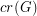ofis the minimum number of crossings in all drawings ofin the plane.

ConjectureKeywords: complete graph; crossing number

## The Crossing Number of the Complete Bipartite Graph ★★★

Author(s): Turan

The crossing numberofis the minimum number of crossings in all drawings ofin the plane.

ConjectureKeywords: complete bipartite graph; crossing number

## The Crossing Number of the Hypercube ★★

Author(s): Erdos; Guy

The crossing numberofis the minimum number of crossings in all drawings ofin the plane.

The-dimensional (hyper)cube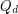is the graph whose vertices are all binary sequences of length, and two of the sequences are adjacent inif they differ in precisely one coordinate.

ConjectureKeywords: crossing number; hypercube

## Drawing disconnected graphs on surfaces ★★

Author(s): DeVos; Mohar; Samal

Conjecture   Letbe the disjoint union of the graphsandand letbe a surface. Is it true that every optimal drawing ofonhas the property thatandare disjoint?

Keywords: crossing number; surface

## Crossing sequences ★★

Author(s): Archdeacon; Bonnington; Siran

Conjecture   Let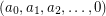be a sequence of nonnegative integers which strictly decreases until.

Then there exists a graph that be drawn on a surface with orientable (nonorientable, resp.) genuswith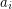crossings, but not with less crossings.

Keywords: crossing number; crossing sequence

## Crossing numbers and coloring ★★★

Author(s): Albertson

We letdenote the crossing number of a graph.

Conjecture   Every graphwithsatisfies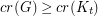.

Keywords: coloring; complete graph; crossing number

## Are different notions of the crossing number the same? ★★★

Author(s): Pach; Tóth

Problem   Does the following equality hold for every graph?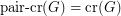The crossing numberof a graphis the minimum number of edge crossings in any drawing ofin the plane. In the pairwise crossing number, we minimize the number of pairs of edges that cross.

Keywords: crossing number; pair-crossing number